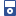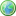Accessibility options:

# Complex Numbers resources

Show me all resources applicable to

### 3GP Mobile Phone (10)Adding and Subtracting Complex Numbers
This mobile phone video explains how complex numbers can be added or subtracted. There is an accompanying leaflet. Sigma resource Unit 4. This resource is released under a Creative Commons license Attribution-Non-Commercial-No Derivative Works and the copyright is held by mathcentre.Division of complex numbers
This mobile phone video explains how to divide complex numbers. Sigma resource Unit 7. This resource is released under a Creative Commons license Attribution-Non-Commercial-No Derivative Works and the copyright is held by mathcentre.Imaginary numbers and quadratic equations
This mobile phone video shows how the imaginary number i can be used in the solution of some quadratic equations. Sigma resource Unit 2. This resource is released under a Creative Commons license Attribution-Non-Commercial-No Derivative Works and the copyright is held by mathcentre.Motivating the study of complex numbers
This mobile phone download introduces complex numbers by explaining how it is useful to be able to formally write down the square root of a negative number. Sigma resource Unit 1. This resource is released under a Creative Commons license Attribution-Non-Commercial-No Derivative Works and the copyright is held by mathcentre.Multiplying complex numbers
This mobile phone video explains how complex numbers can be multiplied together. There is an accompanying leaflet. Sigma resource Unit 5. This resource is released under a Creative Commons license Attribution-Non-Commercial-No Derivative Works and the copyright is held by mathcentre.The Argand Diagram
This mobile phone video explains how complex numbers can be represented pictorially using an Argand Diagram. Sigma resource Unit 8. This resource is released under a Creative Commons license Attribution-Non-Commercial-No Derivative Works and the copyright is held by mathcentre.The complex conjugate
This mobile phone video explains what is meant by the complex conjugate of a complex number. Sigma resource Unit 6. This resource is released under a Creative Commons license Attribution-Non-Commercial-No Derivative Works and the copyright is held by mathcentre.The modulus and argument of a complex number
This mobile phone video explains how to calculate the modulus and argument of a complex number. Sigma resource Unit 9. This resource is released under a Creative Commons license Attribution-Non-Commercial-No Derivative Works and the copyright is held by mathcentre.The polar form of a complex number
This mobile phone video explains what is meant by the polar form of a complex number. Sigma resource Unit 10. This resource is released under a Creative Commons license Attribution-Non-Commercial-No Derivative Works and the copyright is held by mathcentre.What is a complex number
This mobile phone video explains what is meant by a complex number, and how to find its real and imaginary parts. Sigma resource Unit 3. This resource is released under a Creative Commons license Attribution-Non-Commercial-No Derivative Works and the copyright is held by mathcentre.

### iPOD Video (9)Adding and Subtracting Complex Numbers
This mp4 video explains how complex numbers can be added or subtracted. There is an accompanying leaflet. Sigma resource Unit 4. This resource is released under a Creative Commons license Attribution-Non-Commercial-No Derivative Works and the copyright is held by mathcentre.Division of complex numbers
This mp4 video explains how to divide complex numbers. Sigma resource Unit 7. This resource is released under a Creative Commons license Attribution-Non-Commercial-No Derivative Works and the copyright is held by mathcentre.Imaginary numbers and quadratic equations
This mp4 video shows how the imaginary number i can be used in the solution of some quadratic equations. Sigma resource Unit 2. This resource is released under a Creative Commons license Attribution-Non-Commercial-No Derivative Works and the copyright is held by mathcentre.Motivating the study of complex numbers
This mp4 video introduces complex numbers by explaining how it is useful to be able to formally write down the square root of a negative number. Sigma resource Unit 1. This resource is released under a Creative Commons license Attribution-Non-Commercial-No Derivative Works and the copyright is held by mathcentre.Multiplying complex numbers
This mp4 video explains how complex numbers can be multiplied together. There is an accompanying leaflet. Sigma resource Unit 5. This resource is released under a Creative Commons license Attribution-Non-Commercial-No Derivative Works and the copyright is held by mathcentre.The Argand Diagram
This mp4 video explains how complex numbers can be represented pictorially using an Argand Diagram. Sigma resource Unit 8. This resource is released under a Creative Commons license Attribution-Non-Commercial-No Derivative Works and the copyright is held by mathcentre.The complex conjugate
This mp4 video explains what is meant by the complex conjugate of a complex number. Sigma resource Unit 6. This resource is released under a Creative Commons license Attribution-Non-Commercial-No Derivative Works and the copyright is held by mathcentre.The modulus and argument of a complex number
This mp4 video explains how to calculate the modulus and argument of a complex number. Sigma resource Unit 9. This resource is released under a Creative Commons license Attribution-Non-Commercial-No Derivative Works and the copyright is held by mathcentre.What is a complex number
This mp4 video explains what is meant by a complex number, and how to find its real and imaginary parts. Sigma resource Unit 3. This resource is released under a Creative Commons license Attribution-Non-Commercial-No Derivative Works and the copyright is held by mathcentre.

### Quick Reference (10)Adding and Subtracting Complex Numbers
This leaflet explains how complex numbers can be added or subtracted. There are accompanying videos. Sigma resource Unit 4.Division of complex numbers
This leaflet explains how to divide complex numbers. Sigma resource Unit 7. There are accompanying videos.Imaginary numbers and quadratic equations
This leaflet shows how the imaginary number i can be used in the solution of some quadratic equations. Sigma resource Unit 2.Motivating the study of complex numbers
This unit introduces complex numbers by explaining how it is useful to be able to formally write down the square root of a negative number. Sigma resource Unit 1.Multiplying complex numbers
This leaflet explains how complex numbers can be multiplied together. There are accompanying videos. Sigma resource Unit 5.The Argand Diagram
This leaflet explains how complex numbers can be represented pictorially using an Argand Diagram. There are accompanying videos. Sigma resource Unit 8.The complex conjugate
This leaflet explains what is meant by the complex conjugate of a complex number. There are accompanying videos. Sigma resource Unit 6.The modulus and argument of a complex number
This leaflet explains how to calculate the modulus and argument of a complex number. There are accompanying videos. Sigma resource Unit 9.The polar form of a complex number
This leaflet explains what is meant by the polar form of a complex number. There are accompanying videos. Sigma resource Unit 10.What is a complex number
This leaflet explains what is meant by a complex number, and how to find its real and imaginary parts. Sigma resource Unit 3.

### Test Yourself (1)Maths EG
Computer-aided assessment of maths, stats and numeracy from GCSE to undergraduate level 2. These resources have been made available under a Creative Common licence by Martin Greenhow and Abdulrahman Kamavi, Brunel University.

### Video (10)Adding and Subtracting Complex Numbers
This video explains how complex numbers can be added or subtracted. There is an accompanying leaflet. Sigma resource Unit 4. This resource is released under a Creative Commons license Attribution-Non-Commercial-No Derivative Works and the copyright is held by mathcentre.Division of complex numbers
This video explains how to divide complex numbers. Sigma resource Unit 7. This resource is released under a Creative Commons license Attribution-Non-Commercial-No Derivative Works and the copyright is held by mathcentre.Imaginary numbers and quadratic equations
This video shows how the imaginary number i can be used in the solution of some quadratic equations. Sigma resource Unit 2. This resource is released under a Creative Commons license Attribution-Non-Commercial-No Derivative Works and the copyright is held by mathcentre.Motivating the study of complex numbers
This video introduces complex numbers by explaining how it is useful to be able to formally write down the square root of a negative number. Sigma resource Unit 1. This resource is released under a Creative Commons license Attribution-Non-Commercial-No Derivative Works and the copyright is held by mathcentre.Multiplying complex numbers
This video explains how complex numbers can be multiplied together. There is an accompanying leaflet. Sigma resource Unit 5. This resource is released under a Creative Commons license Attribution-Non-Commercial-No Derivative Works and the copyright is held by mathcentre.The Argand Diagram
This video explains how complex numbers can be represented pictorially using an Argand Diagram. Sigma resource Unit 8. This resource is released under a Creative Commons license Attribution-Non-Commercial-No Derivative Works and the copyright is held by mathcentre.The complex conjugate
This video explains what is meant by the complex conjugate of a complex number. There is an accompanying leaflet. Sigma resource Unit 6. This resource is released under a Creative Commons license Attribution-Non-Commercial-No Derivative Works and the copyright is held by mathcentre.The modulus and argument of a complex number
This video explains how to calculate the modulus and argument of a complex number. Sigma resource Unit 9. This resource is released under a Creative Commons license Attribution-Non-Commercial-No Derivative Works and the copyright is held by mathcentre.The polar form of a complex number
This video explains what is meant by the polar form of a complex number. Sigma resource Unit 10. This resource is released under a Creative Commons license Attribution-Non-Commercial-No Derivative Works and the copyright is held by mathcentre.What is a complex number
This video explains what is meant by a complex number, and how to find its real and imaginary parts. Sigma resource Unit 3. This resource is released under a Creative Commons license Attribution-Non-Commercial-No Derivative Works and the copyright is held by mathcentre.

Website design by Pink Mayhem, Leicester